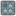• # I have no idea how to start solving this mission.

## Question related to missionMoore Neighbourhood

I originally had something like this:

``` grid = ((1, 0, 0, 1, 0), (0, 1, 0, 0, 0), (0, 0, 1, 0, 1), (1, 0, 0, 0, 0), (0, 0, 1, 0, 0))
count = 0
row = 1
col = 2

if grid[row][col+1] == 1:
count += 1
if grid[row][col-1] == 1:
count += 1
if grid[row+1][col] == 1:
count +=1
if grid[row-1][col] == 1:
count +=1
if grid[row+1][col-1] == 1:
count +=1
if grid[row-1][col-1] == 1:
count +=1
if grid[row+1][col+1] == 1:
count +=1
if grid[row-1][col+1] == 1:
count +=1
return count
```

I get through the first two problem checks fine but fail miserably on the third. I get an 'index out of range' error. When I use exceptions, my count is too high because row[-1] checks the last index. When row or col = 0 I don't want the last index to be checked. The internet has been no help. How can I dynamically check for when the ranges get smaller? What am I missing?10